# Mid-Point Theorem

Go back to  'Triangles-Quadrilaterals'

## What is the Midpoint Theorem?

The Midpoint Theorem says that the line segment joining the midpoints of any two sides of a triangle is parallel to the third side and equal to half of the third side. Consider an arbitrary triangle, $$\Delta ABC$$. Let D and E be the midpoints of AB and AC. Suppose that you join D and E: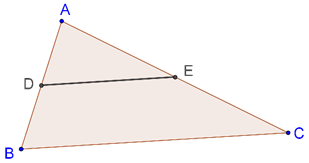The Midpoint Theorem says that DE will be parallel to BC and equal to exactly half of BC.

## Visualise the Midpoint Theorem

In the simulation below, drag the vertices of the triangle to see the Midpoint Theorem in action. DE is always parallel to BC, and equal to exactly half of BC:

## Proof of the Midpoint Theorem

Proof: Through C, draw a line parallel to BA, and extend DE such that it meets this parallel at F, as shown below: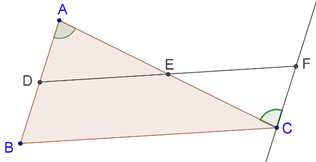Compare $$\Delta AED$$ with $$\Delta CEF$$:

1. AE = EC (E is the midpoint of AC)

2. $$\angle DAE$$ = $$\angle FCE$$ (alternate interior angles)

3. $$\angle DEA$$ = $$\angle FEC$$ (vertically opposite angles)

By the ASA criterion, the two triangles are congruent. Thus, DE = EF and AD = CF. But AD is also equal to BD, which means that BD = CF (also, BD || CF by our construction). This implies that BCFD is a parallelogram. Thus,

1. DF || BC è DE || BC

2. DE = EF = ½(DF) = ½(BC) èDE = ½(BC)

This completes our proof. Will the converse of this theorem hold? Yes, it will, and the proof of the converse is presented next.

### Converse of the Midpoint Theorem

The Converse of the Midpoint Theorem says that the line drawn through the midpoint of one side of a triangle which is parallel to another side will bisect the third side. Consider a triangle ABC, and let D be the midpoint of AB. A line through D parallel to BC meets AC at E, as shown below: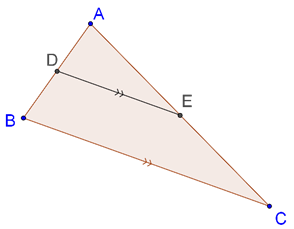The Converse of the Midpoint Theorem says that E is the midpoint of AC.

### Proof of the Converse of the Midpoint Theorem

Proof: Suppose that E is not the midpoint of AC. Let F be the midpoint of AC. Join D to F, as shown below: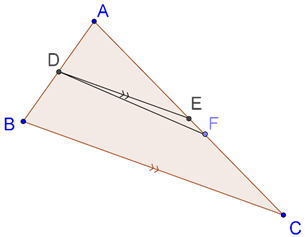By the Midpoint Theorem, DF || BC. But we also have DE || BC. This cannot happen because through a given point (in this case, D), exactly one parallel can be drawn to a given line (in this case, BC).

Thus, E must be the midpoint of AC. This completes our proof.

### Joining the midpoints the sides of a triangle

An interesting consequence of the midpoint theorem is that if we join the midpoints of the three sides of any triangle, we will get four (smaller) congruent triangles, as shown in the figure below: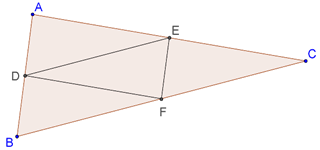We have:

$$\Delta ADE$$ ≡ $$\Delta FED$$ ≡ $$\Delta BDF$$ ≡ $$\Delta EFC$$

Proof: Consider the quadrilateral DEFB. By the midpoint theorem, we have:

1. DE = ½ BC = BF

2. DE || BF

Thus, DEFB is a parallelogram, which means that $$\Delta FED$$ ≡ $$\Delta BDF$$.Similarly, we can show that AEFD and DECF are parallelograms, and hence all the four triangles so formed are congruent to each other (make sure that when you write the congruence relation between these triangles, you get the order of the vertices correct).

### Solved examples on the Midpoint Theorem

Example 1. Consider a triangle ABC, and let D be any point on BC. Let X and Y be the midpoints of AB and AC.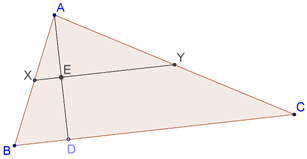Show that XY will bisect AD.

Solution. By the midpoint theorem, XY || BC. Now, consider $$\Delta ABD$$. The segment XE is parallel to the base BD, and X is the midpoint of AB. By the converse of the midpoint theorem, E must be the midpoint of AD.

Thus, XY bisects AD.

Example 2. Prove that if three parallel lines make equal intercepts on one transversal, then they will make equal intercepts on any other transversal as well.

Solution. Let us first understand the problem better. Consider three lines and two transversals, as shown below: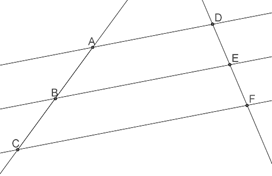Suppose that the intercepts on the left transversal are equal, that is, AB = BC. We then have to prove that the intercepts on the right transversal will also be equal, that is, DE = EF.

To prove this, join A to F: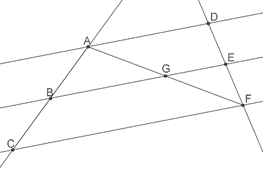Consider $$\Delta ACF$$. Since B is the midpoint of AC and BG || CF, the (converse of the) midpoint theorem tells us that G is the midpoint of AF.

Now, consider $$\Delta AFD$$. We have shown that G is the midpoint of AF. Also, GE || AD.Thus, the (converse of the) midpoint theorem tells us that E must be the midpoint of FD. In other words, DE = EF.

Example 3. Consider a parallelogram ABCD. E and F are the midpoints of AB and CD respectively. Show that the line segments AF and EC trisect the diagonal BD.

Solution. Consider the following figure: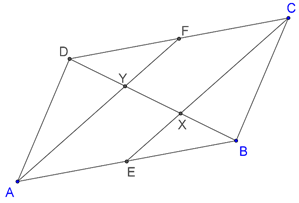We have to show that

BX = XY = YD = BD/3

First of all, we note that AECF is a parallelogram (why?), and thus, EC || AF. Now, consider $$\Delta BAY$$: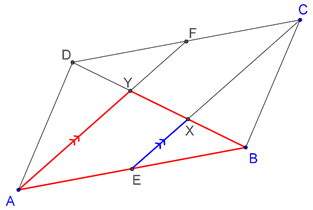Since E is the midpoint of AB, and EX || AY, the (converse of the) midpoint theorem tells us that X is the midpoint of BY, which means that BX = XY. Similarly, we can prove that XY = YD. Thus,

BX = XY = YD = BD/3